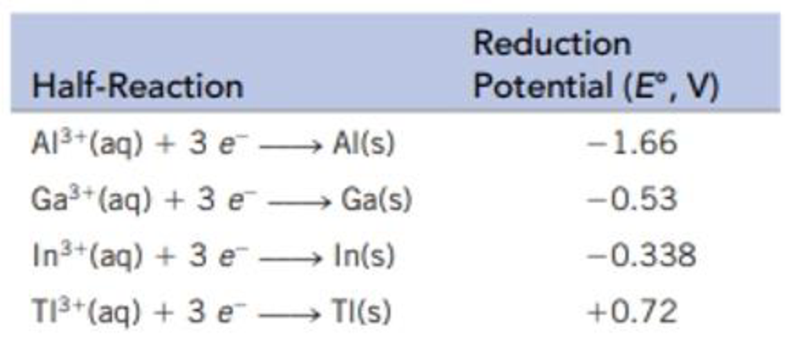Chapter 21, Problem 127SCQ

Chapter
Section
Textbook Problem

The reduction potentials for the Croup 3A metals, E°, are given below. What trend or trends do you observe in these data? What can you learn about the chemistry of the Group 3A elements from these data?Interpretation Introduction

Interpretation:

To observe the trend in the given data and comment on the chemistry of group 3A elements.

Concept introduction:

Reduction potential can be defined as the tendency of an  element to get reduced by gain of electrons. More negative the reduction potential, stronger is the reducing agent.

Down the group the reduction potential increases as the elements prefer to be present in lower oxidation state due to inert pair effect. For example, in group 3A elements, aluminium tends to stay in +3 oxidation number but thallium is more stable in +1 oxidation number.

Explanation

The value of reduction potential is increasing down the group which suggests the reducing ability is decreasing down the group.  The reducing ability is more if the reduction potential has a negative value.

From the given table, it is observed that there is a major drop in value as we move from aluminium to gallium...

Still sussing out bartleby?

Check out a sample textbook solution.

See a sample solution

The Solution to Your Study Problems

Bartleby provides explanations to thousands of textbook problems written by our experts, many with advanced degrees!

Get Started